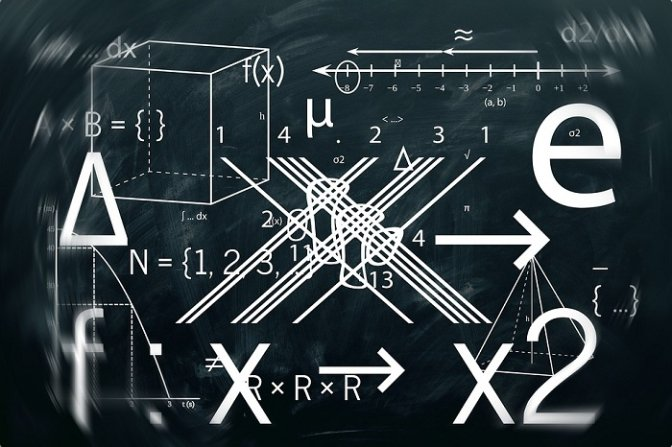The Ultimate Guide to Angles in the World of Mathematics

The angle will always be formed whenever two rays will be joining each other at a particular common point this particular common point is known as nude or vertex. The two rays will be known as the arms of the angle and there is a specific symbol to represent the angles. The angle is normally measured into degrees with the help of a protractor and there are different types of values as well as different types of angles in the whole world of mathematics. The angle is considered to be the geometrical shapes that have been constructed by formulating two rays that will join each other at the endpoints and the angle will also be represented by the three letters of the shape that will help in dividing the angle where the middle letter will be defining the vertex. The angle measurement will always be undertaken most commonly into the degrees only so that people can deal with the things very efficiently.Following are some of the very basic types of angles available in the world of mathematics:

1. The acute angle will be the one that will be lying between 0° and 90°
2. The obtuse angle will be one that will be between 90° and 180°
3. The right angle will be exactly equal to a 90 degree
4. The straight angle will be exactly equal to 180°
5. Reflex angle will be greater than 180° and less than 360°
6. Full rotation will be a complete rotation of angle equal to 360°

The interior angles in the case of the polygon have to be perfectly clear in the minds of kids and the kids also have to have a good idea about the exterior angles as well. The interior angles will be the ones that will be lying inside the polygon or any kind of close shape that will be having sides and angles. The exterior angles will be formed outside the shape between any kind of side or line extended from the adjacent sides.

The positive and negative angles are another very important category in the world of mathematics and the positive angles will be the ones that will be measured in the anticlockwise direction and the negative angle will be measured into the clockwise direction in the whole process.

Following are some of the basic parts of the angles:

• Vertex: This will be the corner point of the angle and will be known as the vertex and this will be the point at which to rays will be meeting each other
• Arms: These will be the two sides of the angle that have been joint at the end common point.
• Initial side: This is known as the reference line and all the measurements will be done taking this particular line as the basic reference.
• Terminal side: This is the side up to which the angle measurement will be done in the whole process

To measure anything in this particular world people always need to have a clear-cut idea about the measurement of angle and for this purpose being clear about the degrees of angles is very much important in the whole process. This is considered to be the best possible method of measuring the angles throughout the process so that kids never face any kind of hassle at any point in time and are very much capable of making the right decisions throughout the process. Another very important measure of an angle is the radian which will always help in making sure that people will be having a numerical value of the measurement of the angle in the whole process.

Hence, having a good command over all these kinds of types of angles is very much important for the people but this is only possible when people will be depending upon Cuemath so that they have a good command over the whole thing without any kind of hassle element in the whole process.

Recommended For You